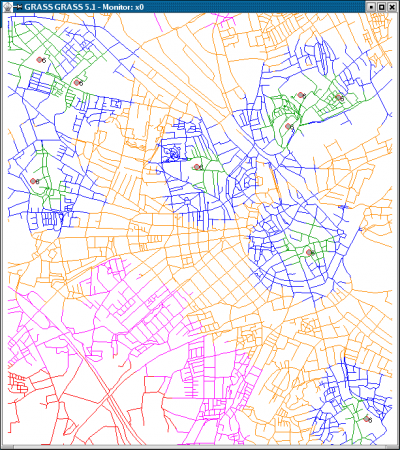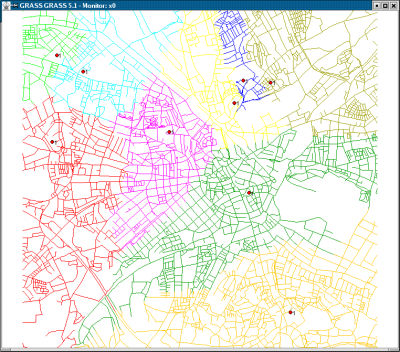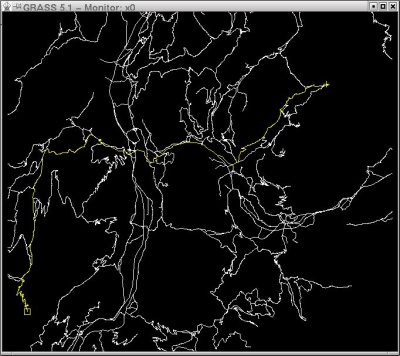# Vector network analysis

GRASS GIS provides support for vector network analysis using the DGlib Directed Graph Library.

GRASS GIS 7 has WxGUI Vector Network Analysis Tool front-end, which supports some of the vector network analysis modules.

## Implemented algorithms

The following algorithms have been implemented:

Vector directions (directed graph) are defined by the digitizing direction (a-->--b). You can navigate either omnidirectionally or differently in each directions as both directions are supported. Network modules provide parameters to assign attribute columns to the forward and backward direction. To see how a vector is directed, use the "display" parameter of d.vect (set display=dir). Use Turntable to assign costs to turns on the network.

## Common parameters

• input - This is the name of input vector map or data source for direct OGR access.
• output - This is the name for output vector map.
• type - This parameter defines arc type, which can be line or boundary.
• alayer - This parameter is a number and defines the arc layer. Vector features can have category values in different layers. This number determines which layer to use. When used with direct OGR access this is the layer name.
• nlayer - This parameter is a number and defines the node layer. Vector features can have category values in different layers. This number determines which layer to use. When used with direct OGR access this is the layer name.
• afcolumn - This is name of the cost column for moving in forward direction or forward and backward directions together.
• abcolumn - This is name of the cost column for moving in backward direction.
• ncolumn - This is name of the cost column for moving through nodes.

If you are not familiar with layers concept in GRASS see Vector Database Management.

## Screenshots

### GRASS GIS 7 screenshotsv.net.iso - Split net to bands between cost isolines (direction from centre). Costs of centre node are used in calculation.v.net.alloc - Allocates subnets for nearest centres (direction from centre). Costs of centre node are used in calculation.d.path - Find shortest path for selected starting and ending node.